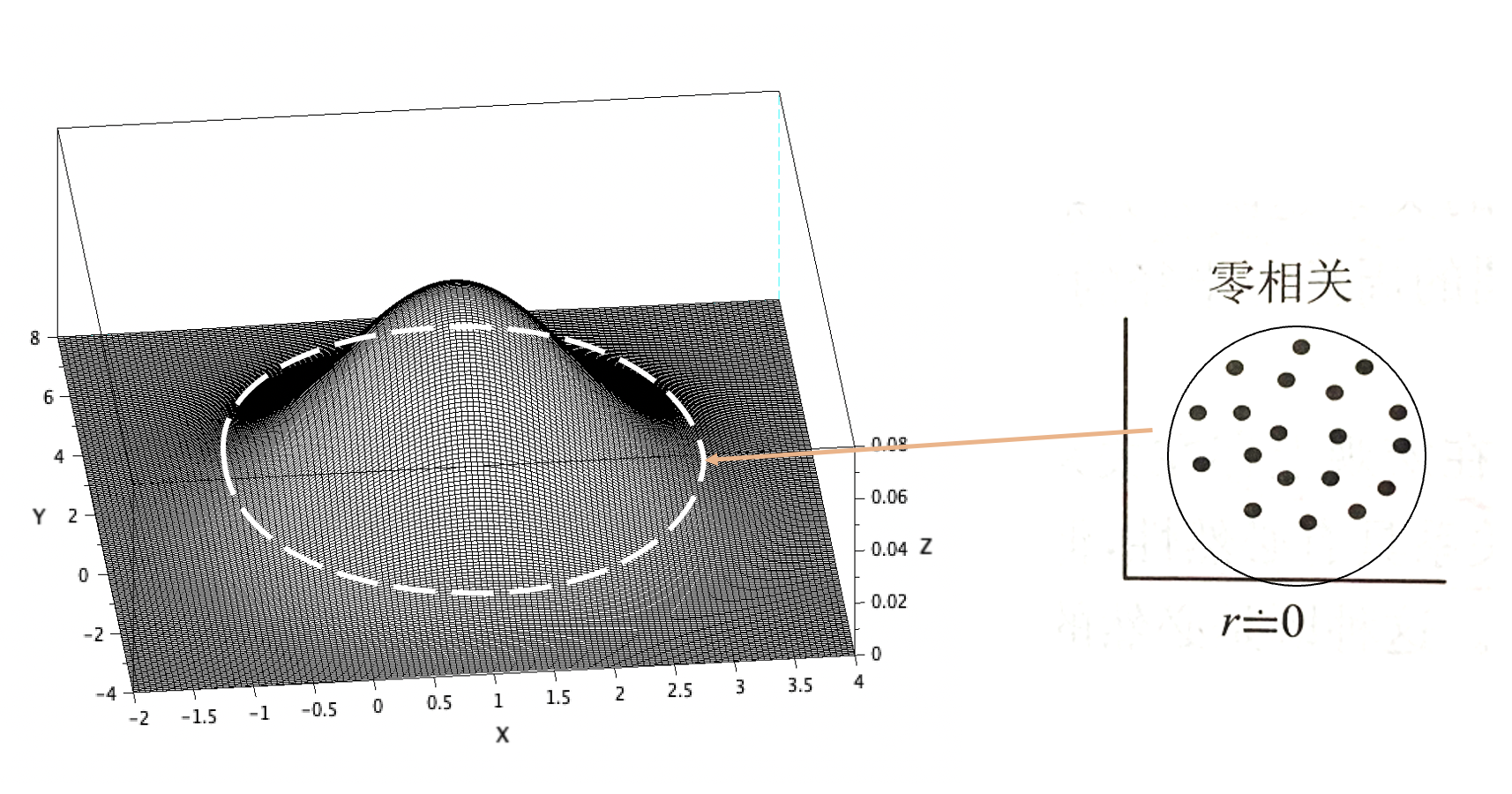V1

2022/08/24阅读：14主题：嫩青

# 【卫生统计学】直线回归的余弦相似度理解角度

## 从余弦相似度理解相关系数

（图1）

### 3. 更直观的感受相关系数

1. 当相关系数r设定为0.01时：（图2，r=0.01）
2. 当相关系数r设定为0.9时：
（图3，r=0.9）

#Scilab 3D normal plot code:u=[1,2];d=[1,2];r=0.9; #设定总体相关系数Rhopi = 3.1415926 #在Matlab中省去此行x=u(1)-3*d(1):0.05:u(1)+3*d(1);y=u(2)-3*d(2):0.05:u(2)+3*d(2);[X,Y]=meshgrid(x,y);part1=(2*pi*d(1)*d(2)*sqrt(1-r^2))^(-1);part2=-1/(2*(1-r^2));partx=(X-u(1)).^2./d(1)^2;party=(Y-u(2)).^2./d(2)^2;partxy=2*r.*(X-u(1)).*(Y-u(2))./(d(1)*d(2));z=part1*exp(part2*(partx-partxy+party));mesh(X,Y,z)

### 参考资料V1